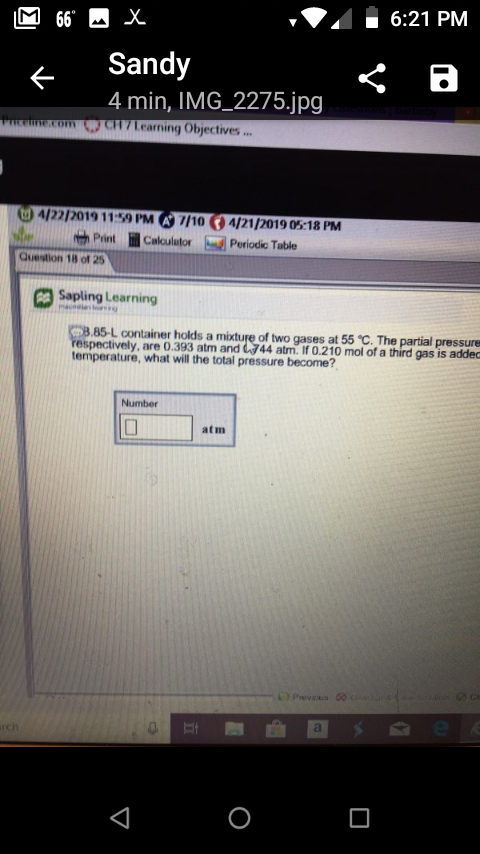6:21 PMSandy4 min, IMG_2275.jpgecom CH7Learning Objectives...t", 帅PrintQuestion 18 of 25lil Calculatorbsd Periodic Table3 Sapling Learning3.85-L container holds a mixture of two gases at 55 ℃. Theespectively, are 0.393 atm and 044 atm. If 0.210 mol of a third gas is addecpressuretemperature, what will the total pressure become?Numberatm

Questionhelp_outlineImage Transcriptionclose6:21 PM Sandy 4 min, IMG_2275.jpg ecom CH7Learning Objectives... t", 帅Print Question 18 of 25 lil Calculator bsd Periodic Table 3 Sapling Learning 3.85-L container holds a mixture of two gases at 55 ℃. The espectively, are 0.393 atm and 044 atm. If 0.210 mol of a third gas is addec pressure temperature, what will the total pressure become? Number atm fullscreen
Step 1

An ideal gas refers to a gas which is composed of particles that move randomly  just like all other gas particles , with the difference that when the particles of an ideal gas collide with each other , these collisions between the gaseous molecules are supposed to be perfectly elastic that means energy of none of these particles would have been destroyed during the collisions.

Step 2

Ideal gas equation is obtained after the combination of all the gas laws such as Boyle’s law , Charles law and Avogadro’s law and the equation so obtained is known as ideal gas equation.

Step 3

Substituting these values in the above equation we get

Hence the...

Want to see the full answer?

See Solution

Want to see this answer and more?

Our solutions are written by experts, many with advanced degrees, and available 24/7

See Solution
Tagged in

Physical Chemistry